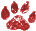# The Rsa Encode Decode Method

 Namespace: WIN_COM_API
The RSA encode-decode method

RSA is a method used to encrypt-decrypt messages in a very secure way.

It s based on the fact that if we have converted our plaintext into block of numbers according to an arbitrary scheme (N1,N2,....,Ns) Ni < n then the sender encodes the Message with an encrypting number e in the following way
`Ni^e (mod n)`
Where `^` means exponention.
andd the receiver decodes the message with a decrypting number d as follows:
`(Ni^e)^d (mod n)`
`e` and `d` are chosen so that
`Ni=(Ni**e)**d (mod n)`

The reader is referred to specialized texts such as A course in Number Theory and Cryptography by Neal Koblitz, Springer Verlag.

A fast exponention method (mod n) is at the heart of this algorithm and can be implemented very easily in VFP. Never use raw exponentiation to compute large exponentes. Let's suppose we want to calculate :
`W**e (mod n)`
Where:
`E=2t+1`
The code in VFP is:
```  ln_result=1
For i=1 to t
ln_result=MOD((W**2)*ln_result,n)
Next
ln_FinalResult=MOD(ln_Result*W,n)
```

In this way we always keep ln_result and ln_FinalResult less than n.

Contributors Eduardo Díaz Guerrero
Category Code Samples
( Topic last updated: 2004.06.13 02:58:10 PM )